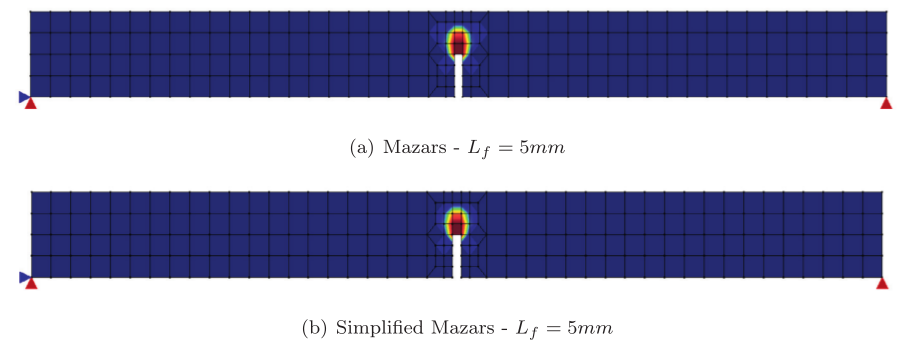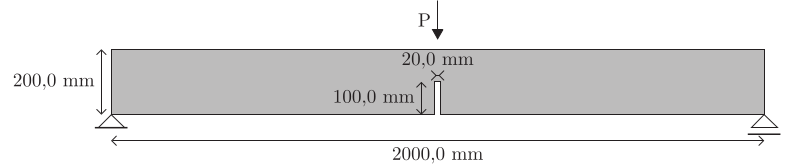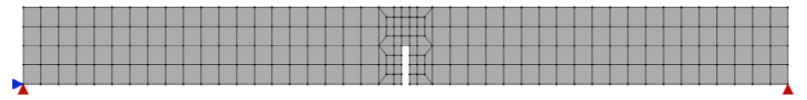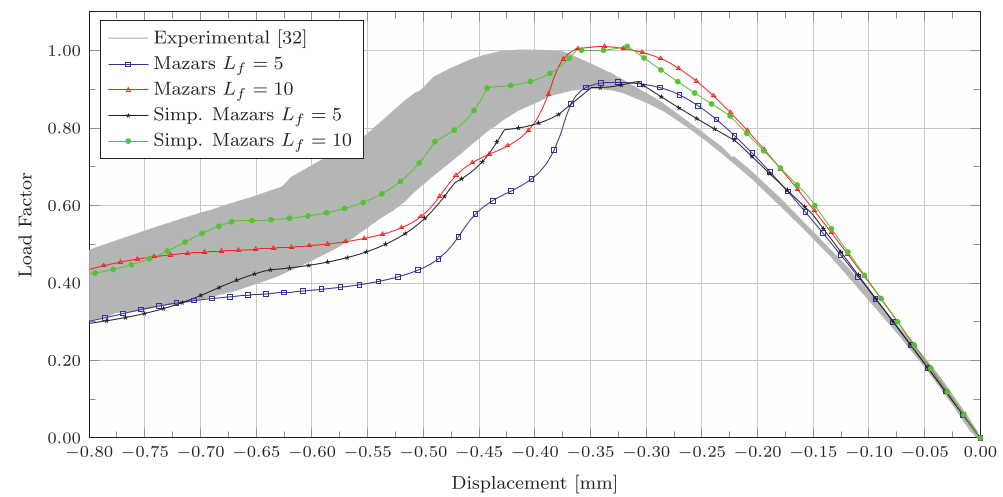# Scalar damage models for micropolar mediaDate:23 Mar, 2017

### Scalar damage models for micropolar media

The software INSANE has been used to model the three-point bending test of a plain concrete beam, analyzed experimentally by Petersson (1981) – Figure 1. A mesh of 204 four nodes quadrilateral finite elements was adopted, with four integration points in each element – Figure 2. The simulation was performed considering a plane stress state. The constitutive models taken into account are adaptations of the classic models of Mazars (Mazars (1984)) and simplified Mazars (de Borst et al. (1999)), proposed by Gori et al. (2017a), and implemented in the constitutive models framework of the software INSANE (Penna (2011), Gori et al. (2017b)). The equilibrium paths relating the load factor with the vertical displacement of the midpoint of the beam are illustrated in Figure 3. These results, obtained combining the constitutive models of Gori et al. (2017a) with an exponential law for the damage evolution, and using different values for the intrinsic length of the micropolar medium, are compared with the experimental values described in Petersson (1981). Figure 4 shows the distribution of damage for both the Mazars and simplified Mazars models, for a loading factor corresponding to a vertical displacement of the midpoint of the beam of -0.48 mm.Figure 1 – GeometryFigure 2 – MeshFigure 3 – Load factor x vertical displacement curvesFigure 4 – Damage distribution

References

• Petersson, P. E., 1981. ‘Crack growth and development of fracture zones in plain concrete and similar materials’. Division of Building materials, Lund Institute of Technology, Lund, Sweden, 28 TVBM-1006.
• Mazars, J., 1984. ‘Application de le mécanique de l’endommagement au comportement non lineaire et à la rupture du béton de structure’. Ph.D. thesis, Univesité Pierre et Marie Curie – Laboratoire de Mécanique et Technologie, Paris, France, 1984.
• de Borst, R. and Gutiérrez, M. A., 1999. ‘A unified framework for concrete damage and fracture models including size effects’. International Journal of Fracture, vol. 95(1-4), pp. 261-277.
• Gori, L., Penna, S. S., Pitangueira, R. L. S., 2017. ‘An enhanced tensorial formulation for elastic degradation in micropolar continua’. Applied Mathematical Modelling, vol. 41, pp. 299-315.
• Penna, S. S., 2011. Formulação multipotencial para modelos de degradação elástica: Unificação teórica, proposta de novo modelo, implementação computacional e modelagem de estruturas de concreto. Ph.D. thesis, Universidade Federal de Minas Gerais, Belo Horizonte, MG, Brasil.
• Gori, L., Penna, S. S., Pitangueira, R. L. S., 2017. ‘A computational framework for constitutive modelling’. Computers and Structures, in press.